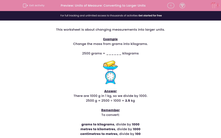# Units of Measure: Converting to Larger Units

In this worksheet, students change the given measurements into larger units.Key stage:  KS 2

Curriculum topic:   Maths and Numerical Reasoning

Curriculum subtopic:   Metric & Imperial

Difficulty level:#### Worksheet Overview

This worksheet is about changing measurements into larger units.

Example

Change the mass from grams into kilograms.

2500 grams = ______ kilogramsThere are 1000 g in 1 kg, so we divide by 1000.

2500 g = 2500 ÷ 1000 = 2.5 kg

Remember

To convert:

grams to kilograms, divide by 1000

metres to kilometres, divide by 1000

centimetres to metres, divide by 100

### What is EdPlace?

We're your National Curriculum aligned online education content provider helping each child succeed in English, maths and science from year 1 to GCSE. With an EdPlace account you’ll be able to track and measure progress, helping each child achieve their best. We build confidence and attainment by personalising each child’s learning at a level that suits them.

Get started